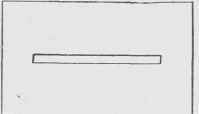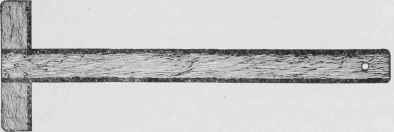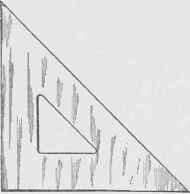In using a very hard lead pencil a light pressure should be used as otherwise the chisel edge will make a deep impression in the paper which cannot be erased. Erasers. What little erasing is necessary in making drawings, should be done with a soft rubber. To avoid erasing the surrounding work some draftsmen use a card in which a slit is cut about 3 inches long and 1/8 to 1/4 inch wide, Fig. 3. An erasing shield of thin metal, Fig. 4, is also very convenient, especially in erasing letters. For cleaning drawings, a sponge rubber or bread crumbs may be used. To clean the drawing scatter dry bread crumbs over it and rub them on the surface with the hand.Fig. 2. Pencil Sharpened to a Chisel Point.Fig. 3. Erasing Shield.Fig. 4. Metal Erasing Shield.

T=Square. The T-square, which gets its name from its general shape, consists of a thin straight-edge, the blade, with a short piece, the head, fastened at right angles to it, Fig. 5. T-squares are usually made of wood, the pear and maple woods being used in the cheaper grades, and the harder woods, like mahogany, with protecting edges or ebony or celluloid, Fig. 6, in the more expensive instruments. The head is designed to fit against the edge of the drawing board, allowing the blade to extend across the surface of the board. It is desirable to have the blade of the T-square make a right angle with the head, but this is not absolutely necessary, if the head is always placed against the left-hand edge of the board, for the lines drawn with the T-square will then be referred to one edge or the board only, and if this edge of the board is straight, the lines will be parallel to each other.Fig. 5. Common T-Square.Fig. G. Mahogany-Bound T-Square.

T-squares are sometimes provided with swiveled heads as it is frequently very convenient to draw lines parallel to each other which are not at right angles to the left-hand edge of the board. To use the T-square in drawing parallel horizontal lines, place the head of the T-square in contact with the left-hand edge of the board, Fig. 7, and draw the pencil along the upper edge of the blade at each new position of the T-square. Only the upper edge should be used as the two edges may not be exactly parallel and straight. In trimming drawings or cutting the paper from the board, always use the lower edge of the T-square so that the upper edge may not be made untrue. For accurate work it is absolutely necessary that the upper edge of the T-square be exactly straight. To test the straightness of the edge two T-squares may be placed together as shown in Fig. 8. However, a lack of contact such as shown in the figure does not prove which edge is crooked, and for this determination a third blade must be used and tried with the two given T-squares successively.Fig. 7. Drawing Parallel Lines.

Triangles. Triangles are made of various substances such as wood, rubber, celluloid, and steel. Wooden triangles are cheap but are likely to warp out of shape; rubber triangles are frequently used, and are, in general, satisfactory; celluloid triangles are extensively used on account of their transparency, which enables the draftsmen to see the work already done even when covered with the triangle.Fig. 8. Testing the Edge of T-Square.Fig. 9. 45° and 30°-60° Triangles.

In using a rubber or celluloid triangle take care that it lies perfectly flat and is hung up when not in use; when allowed to lie on the drawing board with a pencil or an eraser under one corner it will become warped in a short time, especially if the room is hot or the sun happens to strike the triangle.

Triangles from 6 to 8 inches on a side will be found convenient for most work, although there are many cases where a small triangle measuring about 4 inches on a side will be found useful. Every draftsman should have at least two triangles, one having two angles of 45 degrees and one right angle; and the other having angles of 30, 60, and 90 degrees, respectively, Fig. 9.

The value of the triangle depends upon the accuracy of the angles and the straightness of the edges. To test the accuracy of the right angle of a triangle, place the triangle with the lower edge resting on the T-square in position A, Fig. 10. Now draw the line C D, which, if the triangle be true, will be perpendicular to the edge of the T-square. Transfer the triangle to position B, and if the right angle of the triangle is exactly 90 degrees the left-hand edge of the triangle will exactly coincide with the line C D.Fig. 10. Testing a Right Angle (45° Triangle).

To test the accuracy of the 45-degree angles place the triangle with the lower edge resting on the working edge of the T-square, and draw the line E F, Fig. 11. Now without moving the T-square place the triangle so that the other 45-degree angle is in the position occupied by the first. If the two 45-degree angles coincide they are accurate.